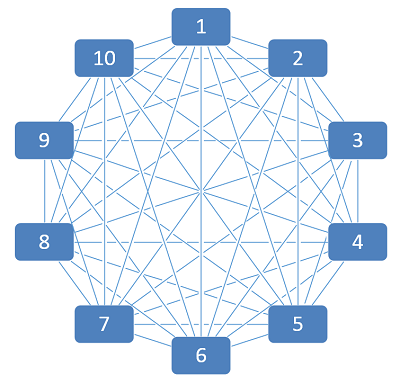# Calculate the Number of Connections Between Objects or People

I was working on some training material and wanted to show how complex ad hoc connections between systems can be. I needed to calculate the number of potential connections between a number of systems, so I had to relearn a formula.

Between 2 systems there is only 1 possible connection.One way to calculate this for more than 2 objects is to add up all the whole numbers between 1 and (n-1) where n is the number of objects.

Connections between 3 systems:
1+2 = 3 possible connectionsConnections between 4 systems:
1+2+3 = 6 possible connections1+2+3+4+5+6+7+8+9 = 45 possible connections

Thankfully, there is a formula to calculate the number of connections between a given number of objects:
n(n-1)
2

Connections between 10 systems:
10(10-1)/2 = 45 possible connectionsConnections between 20 systems:
20(20-1)/2 = 190 possible connectionsAs you can see, the complexity greatly increases with each new objected added to the group. This growing complexity can be overcome in systems design through good Service-Oriented Architecture (SOA).

In what other scenarios would this formula come in handy?
• Possible number of direct flights between a number of cities
• Possible number of exchange rates between different currencies
• Possible number of translators needed within a group representing a number of different languages
• And the classic: Number of communication channels between a number of people in a group

The last example points to why smaller groups often are more productive at communicating and making decisions than larger groups. This is known as the "communications channels formula" in fields like Project Management. Each new person adds (n-1) new communication paths. So:
• 2 people = 1 communication channel
• 3 people = 3 communication channels
• 4 people = 6 communication channels
• 5 people = 10 communication channels
• 10 people = 45 communication channels
• 20 people = 190 communication channels
• 40 people = 780 communication channels

Keep this in mind whenever you are putting together a project team or a governance board!